# Quiz 20: Satellite Communication

The orbital period of the satellite depends on two parameters namely circumference of the orbit, and the velocity of the satellite. The satellite nearer to the earth's surface is in smaller elliptical orbit compared to the satellite that is farther to the earth's surface, since the farther one is in longer elliptical orbit. So generally the distant satellite takes more time to complete one revolution around the earth. The mathematical representation of orbital period of the satellite is,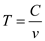Here,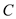is the circumference of the orbit, and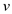is the velocity in meters per second. The circumference of the orbit is,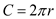Here,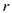is the radius of orbit.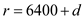Here,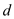is the distance above the earth's surface in km. The velocity of satellite is,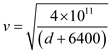When the satellite is in orbit farther from the earth's surface, then it travels with lower speed compared to the satellite in orbit closer to the earth's surface. Because, the satellite in farther orbit takes more time to complete an orbit. If the distance between satellite and earth is more, then circumference becomes more which directly effects the orbital period. If the satellite is farther from the earth surface then the velocity of satellite is less as the distance inversely affects the velocity. Observe this relation from the velocity equation. This velocity in turn effects the period of the orbit for a satellite. Therefore, the orbital period of the distant satellite is much higher compared to the satellite that is nearer to earth's surface.